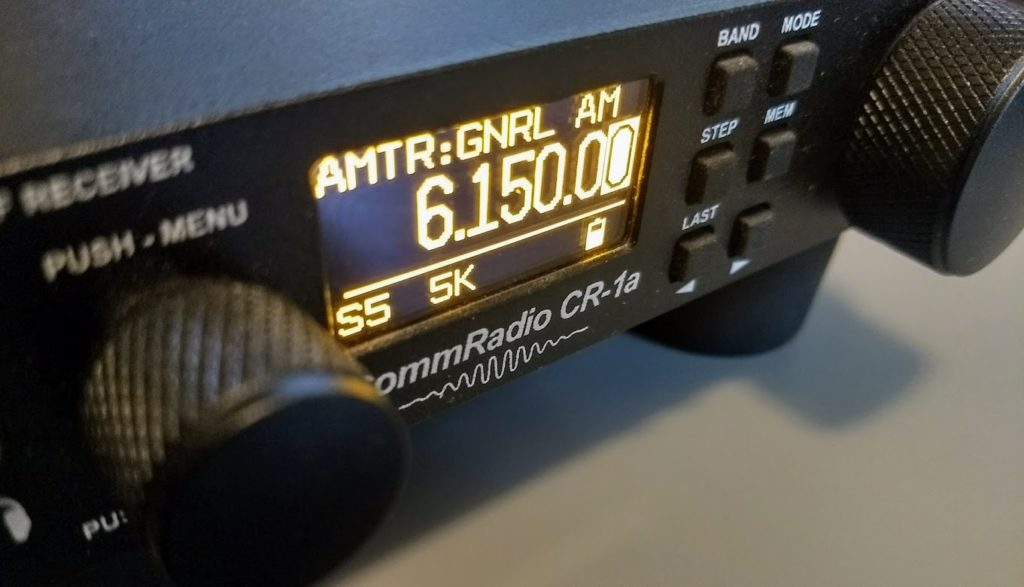# Understanding decibelsMany thanks to SWLing Post contributor, Marty, who shares the following article from Nuts and Volts magazine–a primer on decibels:

The “dee-bee” is everywhere in ham radio, and is used for characterizing everything from antenna performance to nano-sized signals. Learn the decibel (abbreviated as lower-case ‘d’ followed by an upper-case ‘B’ or ‘dB’) and you and your signal will go a long way!

From the ARRL Ham Radio License Manual’s online math tutorials for beginning hams (arrl.org/chpt-2-radio-signal-fundamentals), we introduce the decibel. “You have probably recognized deci as the metric prefix that means one-tenth. The unit we are really talking about here is the bel (a ratio of sound levels named for Alexander Graham Bell), so a decibel is just 1/10th of a bel. We use a decibel instead of a whole bel because the bel represents a rather large change in levels. The dB is a just-perceptible change and more useful as a unit of measurement.” As used in wireless, the decibel is the ratio of two power levels:

dB = 10 log10 (P2/P1)

Note that the dB has no units because it is a ratio. The dB is just a number that describes how much bigger or smaller one quantity is compared to the other. Both quantities themselves must have the same base units, though — watts, for example. If P2 is larger than P1, the dB value is positive, such as for amplifier gain. If P2 is less, the value is negative and represents attenuation or loss. (Somewhat confusingly, it’s common to specify an amount of attenuation as a positive value of dB. For example, “This filter attenuates the signal by 20 dB.”)

## 4 thoughts on “Understanding decibels”

1.mangosman

The answer is all the time.
Hearing is logarithmic so that you can hear the quietest bird tweet to the sound of a jet taking off. So all sound measurements are in dB.
An increase in sound power of 3 dB (2 x power) is just noticeable where as 10 dB (10 times) is about twice as loud.
Radios have amplifiers who’s amplification can be large so for example if the output power is 100 Watts and the input is 1 Watt the amplification is 20 dB
Not mentioned here is the reverse function which is divide the number of dB by 10 then the antilog (in Excel spread sheets it is 10^ ratio.
To reverse the example above if you know the amplification such as 20 dB and you measure the input power of 1 W, then 20/10 = 2. Then in Excel =10^2 = 100 Now the output power is 1 x 100 = 100 W.

Instead of increasing the power you may wish to reduce the power to prevent overloading for example the calculations are identical but the value in dB is negative and the results are fractions of 10.

Other applications
If the impedance or resistance is the same on the input and the output you can use dB = 20 log Voltage out/Voltage in
To reverse the calculation Voltage out/Voltage in = antilog10(dB/20) The antilog is the 10^number I used above.

dB and a reference is a measure of power for example
dBW can be used to quote the power output of a transmitter. (Reference is the Watt)
Why would you want to do this if you double the distance between the transmitter’s aerial and the receiving aerial the signal drops by 6 dB, so you would need to increase the transmitter power by 4 times for the same signal strength. If you keep doing this the drops in signal strength will produce very large numbers very quickly.

dBu, the reference is in microVolts across 75 ohms. This is a common measure of receiver maximum sensitivity. Remember that receivers have automatic gain control which has to reduce the gain as the signal gets stronger, to cope with the varying distance between the transmitter and the receiver for a start.
There is also a reference for measurement of sound which is at the threshold of hearing, but commonly filters are used to match the sensitivity of the ear to various frequencies. The most common version is dBA for quiet sounds and dBC for loud sounds.

The main reason dB is used is to prevent errors when copying down large numbers for example a professional sound system can have a maximum signal to noise ratio of 90 dB which is 1,000,000,000.

1.mangosman

To make it easier for the mathematically challenged, Not only can Excel reverse from dB back to a ratio, to convert to dB, Excel has a formula called log10 so to convert a ratio to dB you type = 10*log10(P1/P2). P1 is one power and P2 another power at a different location or time of measurement.

This site uses Akismet to reduce spam. Learn how your comment data is processed.